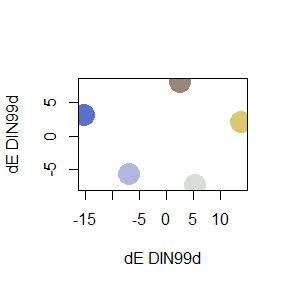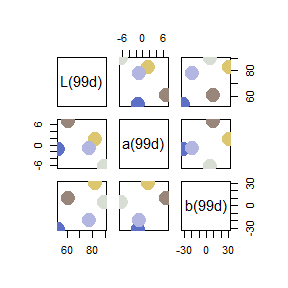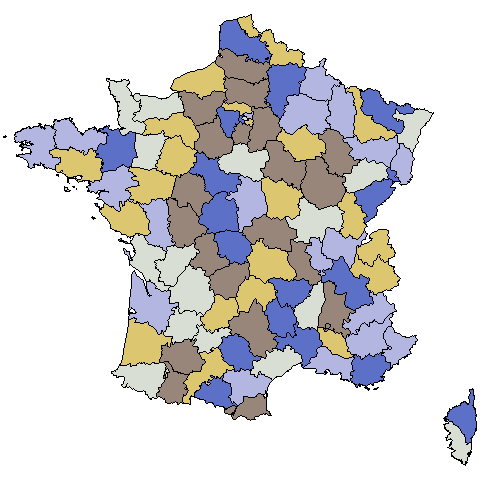# An introduction to qualpalr

## Overview

qualpalr generates distinct qualitative color palettes, primarily for use in R graphics. Given n (the number of colors to generate), along with a subset in the hsl color space (a cylindrical representation of the RGB color space) qualpalr attempts to find the n colors in the provided color subspace that maximize the smallest pairwise color difference. This is done by projecting the color subset from the HSL color space to the DIN99d space. DIN99d is (approximately) perceptually uniform, that is, the euclidean distance between two colors in the space is proportional to their perceived difference.

## Examples

qualpalr relies on one basic function, qualpal(), which takes as its input n (the number of colors to generate) and colorspace, which can be either

• a list of numeric vectors h (hue from -360 to 360), s (saturation from 0 to 1), and l (lightness from 0 to 1), all of length 2, specifying a min and max.
• a character vector specifying one of the predefined color subspaces, which at the time of writing are pretty, pretty_dark, rainbow, and pastels.
library(qualpalr)
pal <- qualpal(n = 5, list(h = c(0, 360), s = c(0.4, 0.6), l = c(0.5, 0.85)))

# Adapt the color space to deuteranomaly of severity 0.7
pal <- qualpal(n = 5, colorspace = "pretty", cvd = "deutan", cvd_severity = 0.7)

The resulting object, pal, is a list with several color tables and a distance matrix based on the DIN99d color difference formula.

pal
#> -------------------------------------
#> Colors in the HSL color space
#>
#>         Hue Saturation Lightness
#> #DCC670  60       0.46      0.61
#> #5C70C8 248       0.48      0.60
#> #99867A 353       0.50      0.62
#> #D9DED5 151       0.48      0.83
#> #B2B6E0 280       0.48      0.78
#>
#>  -------------------------------------
#> DIN99d color difference distance matrix
#>
#>         #DCC670 #5C70C8 #99867A #D9DED5
#> #5C70C8      29
#> #99867A      16      20
#> #D9DED5      15      24      17
#> #B2B6E0      23      15      17      15

Methods for pairs and plot have been written for qualpal objects to help visualize the results.

# Multidimensional scaling plot
plot(pal)

# Pairs plot in the Lab color space
pairs(pal, colorspace = "DIN99d")The colors are most easily used in R by accessing pal$hex library(maps) map("france", fill = TRUE, col = pal$hex, mar = c(0, 0, 0, 0))## Details

qualpal begins by generating a point cloud out of the HSL color subspace provided by the user, using a quasi-random sobol sequence from randtoolbox. Here is the color subset in HSL with settings h = c(-200, 120), s = c(0.3, 0.8), l = c(0.4, 0.9).

The program then proceeds by projecting these colors into the sRGB space.

It then continues projecting the colors, first into the XYZ space, then CIELab (not shown here), and then finally the DIN99d space.

The DIN99d color space (G. Cui et al. 2002) is a euclidean, perceptually uniform color space. This means that the difference between two colors is equal to the euclidean distance between them. We take advantage of this by computing a distance matrix on all the colors in the subset, finding their pairwise color differences. We then apply a power transformation (Huang et al. 2015) to fine tune these differences.

To select the n colors that the user wanted, we proceed greedily: first, we find the two most distant points, then we find the third point that maximizes the minimum distance to the previously selected points. This is repeated until n points are selected. These points are then returned to the user; below is an example using n = 5.

### Color specifications

At the time of writing, qualpalr works only in the sRGB color space with the CIE Standard Illuminant D65 reference white.

## Thanks

Bruce Lindbloom’s webpage has been instrumental in making qualpalr. Thanks also to i want hue, which inspired me to make qualpalr.